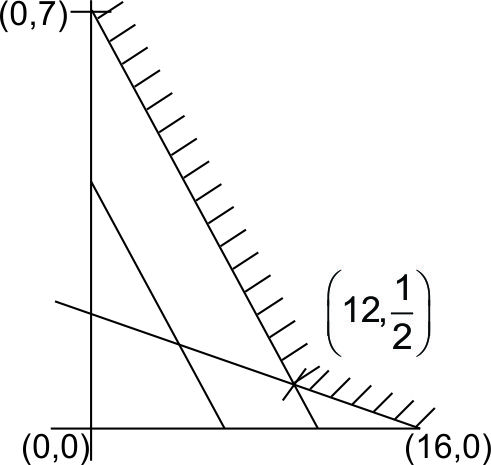# Consider the following linear programming problemMaximizeSubject toFor which values of P is there no solution to this problem? Option 1) P=0 Option 2) P<0 Option 3) P=2 Option 4) P>0

As we learnt in

Decision Variables -

In Z = ax+by, x and y are decision variables.

-for= 0+7 at (0,7)

Z = 16 P + 0 = 16 P at (16, 0)so that forSo far P <O  it has no solution.

Option 1)

P=0

Incorrect

Option 2)

P<0

correct

Option 3)

P=2

Incorrect

Option 4)

P>0

Incorrect

Boost your Preparation for JEE Main 2021 with Personlized Coaching

Exams
Articles
Questions